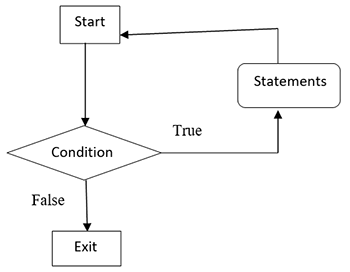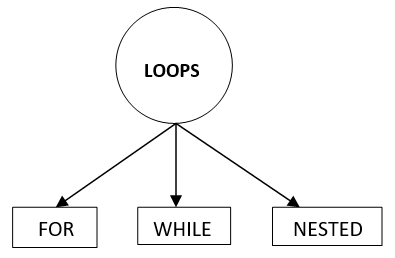Loop in Python | Python Tutorials | Learn Python Online# Loop in Python

2822

What do you mean by a loop in python language?
You can easily execute a single statement or a block of statement multiple times with the help of looping. For entering in any loop there are specified some conditions that are defined at the beginning. Once that condition becomes false, it will stop the execution of the loop and the control exit from the loop.
There are two types of loop i.e. infinite and finite.

Finite loopIn this flowchart, the control comes and check the condition first and if it is true, it will execute the statements present inside the loop and again it go back and check the condition and if again it is true it will again execute the statements and similarly it will continue this process until the condition become false and the moment this condition becomes false, the control will exit from the loop.

Infinite Loop
In an infinite loop, the condition will never be false and the control will never get exit from the loop and the loop will execute unlimited times.
In every language such as C, C++, we have seen the loop is categorized on post-test and pre-test loop. In python, there is no post-test loops.

Type of Loops found in PythonWhile Loop
They are known as the conditional and indefinite loops. These loops will continue iterating until some specific conditions are met. These loops are required when the no of iterations is unknown or in other words, we can say that we do not know the number of times the execution of the statements presents inside the loop are required.

Syntax:
while expression:
statements of while loop body;

A simple program illustrating the while loop in python language

`total=0while total<5:    print("welcome in codingtag python tutorial")    total = total +1`

Output
welcome in codingtag python tutorial
welcome in codingtag python tutorial
welcome in codingtag python tutorial
welcome in codingtag python tutorial
welcome in codingtag python tutorial

For Loop
It is basically applicable when you already know the number of iterations that are required unlike while loop. It provides syntax that consists of three parts i.e. initial value, condition and incrementation which are given below:

Syntax
for <variable> in range:
first statement
second statement
......
statement n

A simple program illustrating For Loop by performing the addition of marks of the student stored in a list

`marks = [6, 5, 3, 8, 4, 13, 5, 4, 11]addition = 0for val in marks:    addition = addition+valprint("The addition of marks of the student is", addition)`

Output
('The addition of marks of the student is', 59)

Nested loop
Python language permits us to use a loop inside another loop i.e. we can easily use for loop inside for loop and while loop inside while loop in python language.

Syntax:
while expression:
while expression:
statements

A simple program illustrating nested while loop

`k = 2while(k < 55):   z = 2   while(z <= (k/z)):      if not(k%z): break      z = z + 1   if (z > k/z) : print k, " is prime"   k = k + 1print "output with the help of nested loop!"`

Output
2 is prime
3 is prime
5 is prime
7 is prime
11 is prime
13 is prime
17 is prime
19 is prime
23 is prime
29 is prime
31 is prime
37 is prime
41 is prime
43 is prime
47 is prime
53 is prime

output with the help of nested loop!Share:## Get a .COM for just \$6.98

Secure Domain for a Mini Price